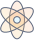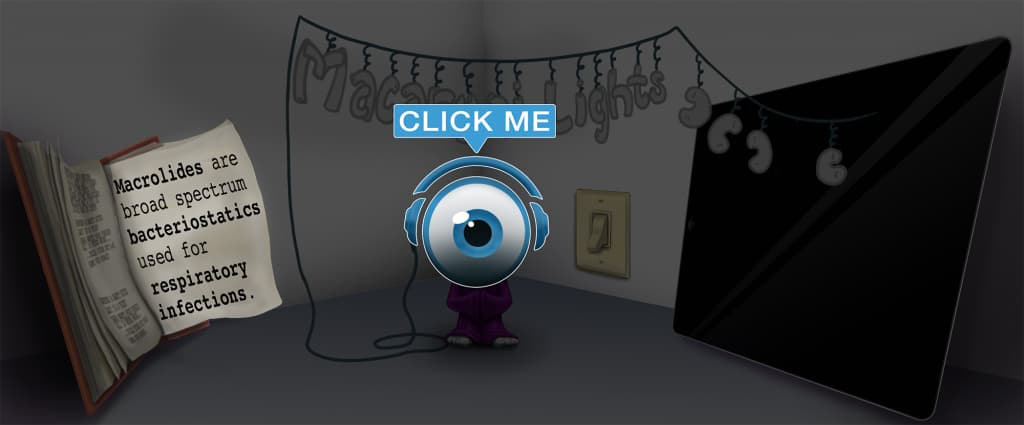# Master Odds Ratio (OR) with Picmonic for Medicine

## With Picmonic, facts become pictures. We've taken what the science shows - image mnemonics work - but we've boosted the effectiveness by building and associating memorable characters, interesting audio stories, and built-in quizzing.

### Odds Ratio (OR)

Odds-dice RatioPicmonic
The odds ratio is a way to measure association between individuals who have a disease and those that do not have disease and how much the presence or absence of a risk factor influences the outcome. It is most commonly derived from a case-control study and is calculated by using the values from a 2x2 contingency table in the formula OR = (a/c)/(b/d), or alternatively OR = ad/bc.
10 KEY FACTS
USE
Case Control Studies
Case and Controller

These observational studies look at two groups based on outcome; individuals with disease, or cases, and similar individuals without disease, or controls. Looking retrospectively, the study seeks to identify risk factors common among these groups and then calculate how much a given risk factor weighs on the outcome. This calculation is quantified as the odds ratio.

Measures Association Between an Exposure and an Outcome
Measuring between Exposure to radiation and Healthy or Diseased Outcome

An odds ratio provides the probability that an individual with a disease or specific outcome was exposed to a risk factor at some point in the past.

FORMULA
Odds of Exposure in Diseased (Sick) Group
Odd-dice with Diseased group Exposed to radiation

The numerator of the formula compares the groups of people who have the disease and the odds that the people in these groups were exposed to a risk factor.

a / c
A-apple Divided by C-cat

The numerator of the formula is calculated by dividing those with disease and exposure (a) by those with disease but without exposure (c).

Divided /
Divide

This division step should be carried out last. First, divide the two terms found in the numerator and denominator respectively before dividing them altogether. Alternatively, you may rearrange the formula so that it becomes (a*d)/(b*c).

Odds of Exposure in Healthy Group
Odds-dice with Healthy group Exposed to radiation

The denominator of the formula compares the groups of people who do not have the disease and the odds that the people in these groups were exposed to a risk factor.

b / d
B-bee body-armor Divided by D-dog

The denominator of the formula is calculated by dividing those without disease but with exposure (b) by those without disease or exposure (d).

INTERPRETATION
=1 Exposure does not affect odds
Equal to 1 shows radiation Exposure with No Effect

If the odds ratio is equal to one, then this implies that there is no association between the outcome and exposure, or in other words, exposure is not a risk factor for the outcome.

> 1 Exposure increases disease
Greater-than 1 shows Exposure to radiation causes Up-arrow Disease

When the odds of exposure for those with the outcome (cases) is more than those without the outcome (controls), it implies that exposure may increase the risk of disease.

< 1 Exposure decreases disease
Less-than 1 shows Exposure to radiation removes Down-arrow Disease

When the odds of exposure for those with the outcome (cases) is less than those without the outcome (controls), it implies that exposure may reduce the risk of disease.

Unlock all 10 facts & definitions with Picmonic Free!

## Take the Odds Ratio (OR) Quiz

Picmonic's rapid review multiple-choice quiz allows you to assess your knowledge.

## Picmonic for Medicine CoversMedicine (MD/DO)
1,800+ Videos
21,000+ Facts

## Our Story Mnemonics Increase Mastery and Retention

Memorize facts with phonetic mnemonics

Unforgettable characters with concise but impactful videos (2-4 min each)

### Ace YourMedicine (MD/DO)Classes & Exams with Picmonic:

#### Over 1,120,000 students use Picmonic’s picture mnemonics to improve knowledge, retention, and exam performance.